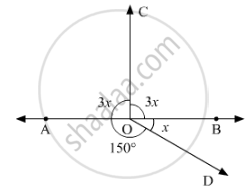# In Below Fig, Determine the Value of X. - Mathematics

In below fig, determine the value of x.#### Solution 1

Since sum of all the angles round a point is equal to 360°.Therefore

⇒ 3x + 3x +150 + x = 360°

⇒ 7x° = 360° -150°

⇒ 7x = 210°

⇒ x = 210/7

⇒ x = 30°

#### Solution 2

In the given figure:AOB is a straight line. Thus,∠AODand  ∠BODform a linear pair.

Therefore their sum must be equal to180°.

We can say that

∠AOD+∠BOD = 180°

It is given that ∠AOD=150°, substituting this value in equation above, we get:

150° + x = 180°

 x = 180° - 150°

  x =30°

Concept: Introduction to Lines and Angles
Is there an error in this question or solution?
Chapter 10: Lines and Angles - Exercise 10.2 [Page 15]

#### APPEARS IN

RD Sharma Mathematics for Class 9
Chapter 10 Lines and Angles
Exercise 10.2 | Q 8 | Page 15
Share